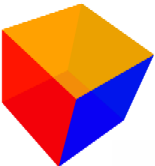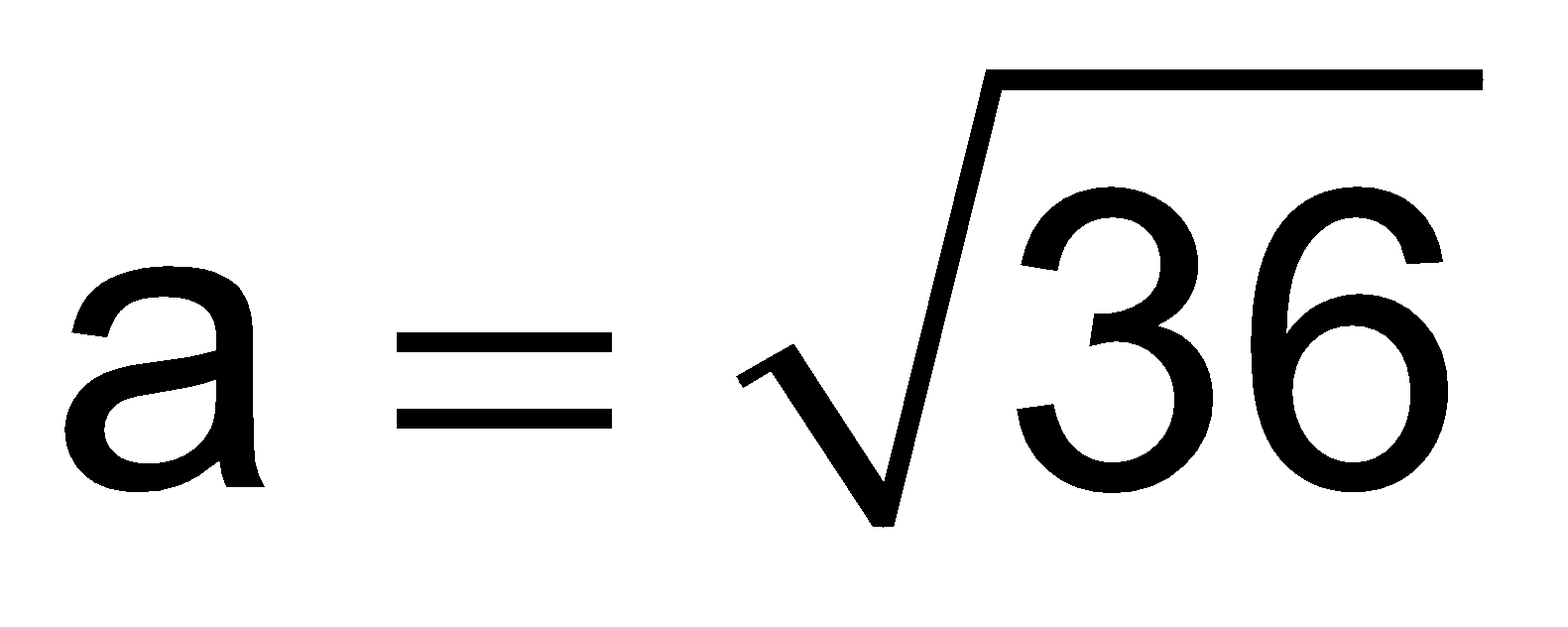# mensuration formulas class 8 cube

## CUBE:

In a solid all three lengths are equal and right angle to each other it is called cube.CubeSolids like ice cubes, sugar cubes, dice, etc., are in the shape of a cube.

For cube having each edge = a units, we have

• Volume a3 cubic units
• Total surface area = 6a2sq units
• Lateral surface area = 4a2sq units

### SOLVED EXAMPLES

question The total surface area of a cube is 216 cm2.Find its volume

Solution: Let each side of the cube be a cm.

Then, the total surface area of the cube =  (6a)2 cm2.

∴ 6a2 = 216 ⇔ a2 = 36 ⇔= 6

Volume of the cube = a3 cubic units

= ( 6× 6 × 6) cm3 = 216 cm3.

question The volume of cube is 4096 cm3.Find its total surface area

Solution: Let each side of the cube be a cm.

Then, the volume of the cube = a3cm3

∴ a3= 4096⇔ a = 16

the total surface area of the cube =  (6a)2 cm2.

= 6×162

=  1536 cm2

## CBSE NCERT Solutions for Class 8 Maths

Notes,worksheet and solved question for Maths class 8

Check your marks in a chapter which you have complited in school from Physics Wallah chapter wise online test just click on the link given below